## Alteryx: Spatial Shortcuts, measuring distances and drawing poly lines

by Laine Caruzca

In Alteryx, you can do some very cool spatial analysis. In this example, I will be talking about how to find distances between two points and how to draw a line between them.

First, I will show you the long way. Then, I will then show you the shortcut.

You can then transfer this to tableau via a shape file (aka shp. File). It is better to use a shape file as this takes up less space/memory. This is the beauty of Alteryx because it can store numerous data points in just one row!

The long way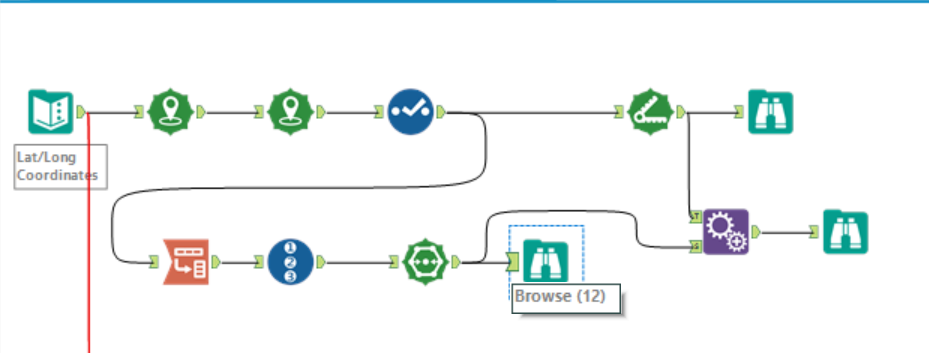• You first need to use the ‘Create Points’ tool to create a spatial point for each data point. In this dataset, we needed to use the tool twice, because we had the longitude and latitude values for 2 points: the start and end points.
• To find the distance, you need to use the ‘Distance’ tool. Select your start and end points and run the workflow. It will then create a new field/column with all the distances.
• To create a polyline between the two points, you need to set up your data in a certain way. The two spatial data points need to be on top of each other, like below: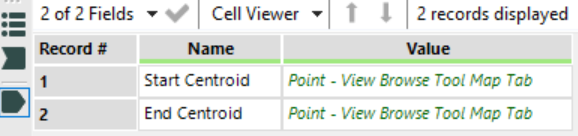• You can then use the ‘Polybuild’ tool to create a line. Under the Build Method, select ‘Sequence Polygon’ and under Sequence Field, select by which fields and order you want to draw your line.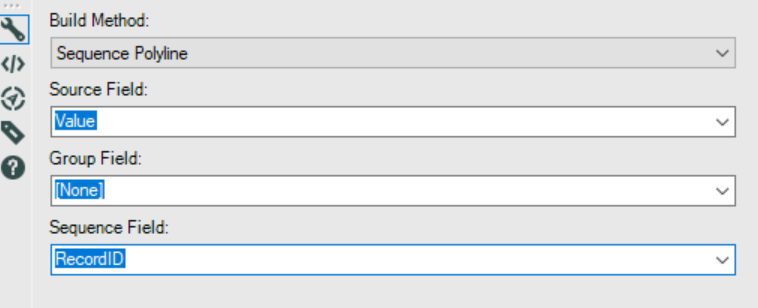• The final step is to use the ‘Append tool’. This is a type of join on Alteryx. I used this tool here because there wasn’t a common field to join them with if I used the normal ‘Join’ tool.

The short wayAs you can see, this is a far shorter way of finding the distance between 2 points and creating a line between them.

Introducing the ‘Formula Tool’, ladies and gents!

With the formula tool, you can basically do anything. I used the following formulas.

TIP: Don’t forget to change your data types!

First, I created the spatial data for the first point, by using the formula above: ‘ST_CreatePoint’.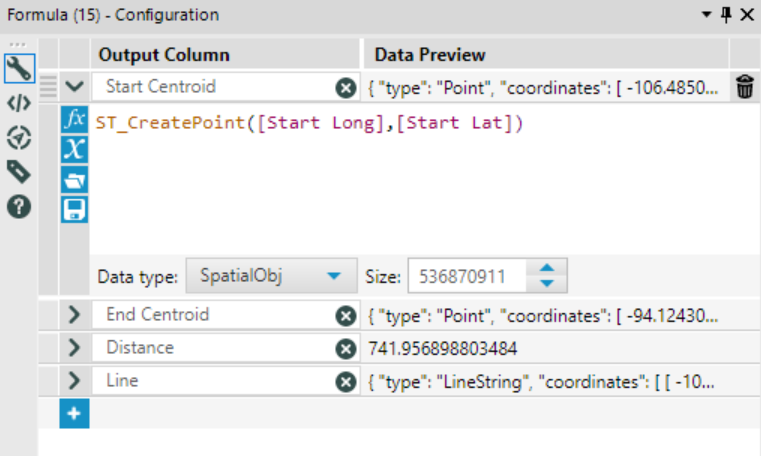Second, I created the spatial data for the second point, as seen below.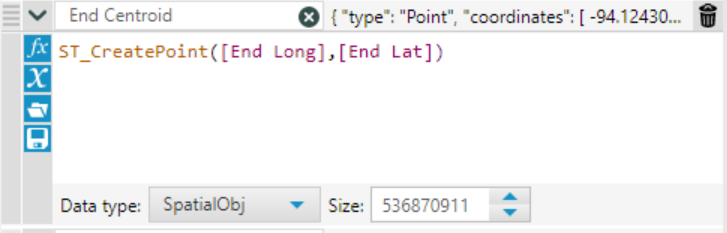Third, I measured the distance between the two points, using the formula below.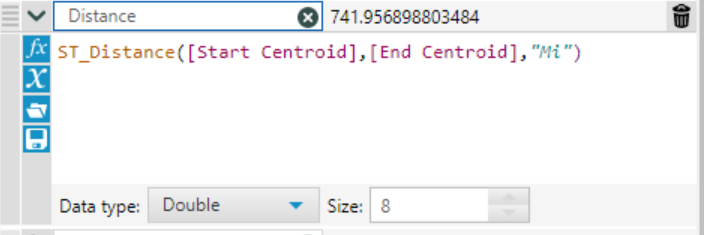Fourth, I created the polyline using the formula below.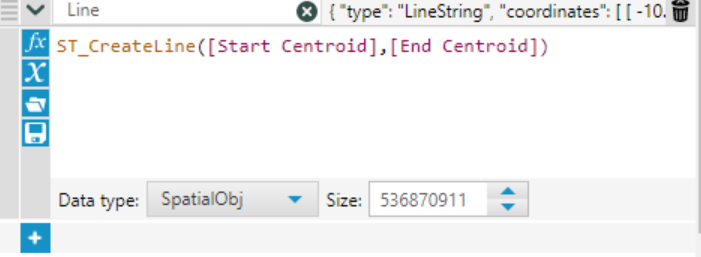After you run the workflow, you end up with the same result!

So there you go! Spatial is definitely the coolest thing I’ve learnt so far, so I hope you guys enjoy exploring with it too!Laine Caruzca

Sun 04 Nov 2018

Sat 18 Aug 2018